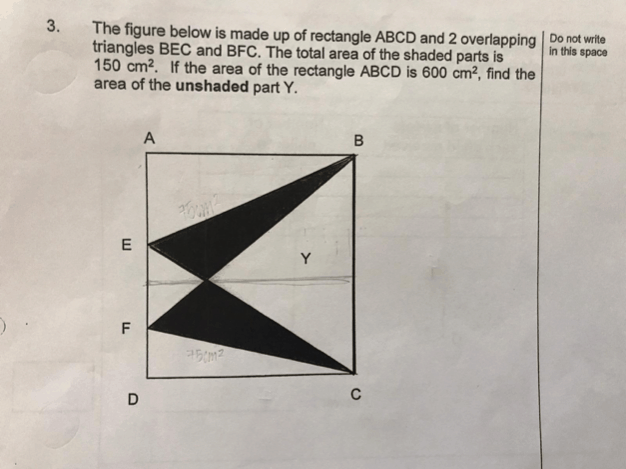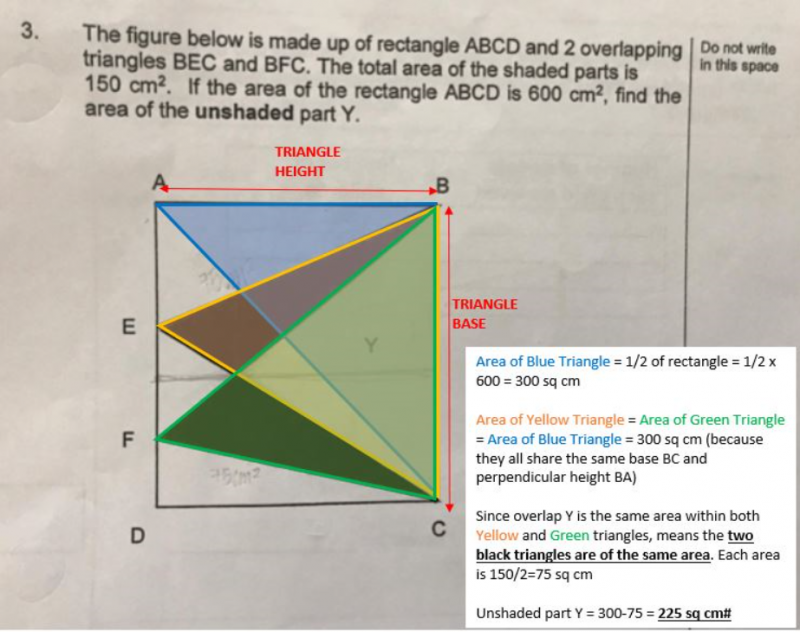# QuestionMay I know anyone can help with the above?  Many thanks in advance.

2 Answers

# AnswerArea of Blue Triangle = 1/2 of rectangle = 1/2 x 600 = 300 sq cm

Area of Yellow Triangle = Area of Green Triangle = Area of Blue Triangle = 300 sq cm (because they all share the same base BC and perpendicular height BA)

Since overlap Y is the same area within both Yellow and Green triangles, means the two black triangles are of the same area. Each area is 150/2=75 sq cm

Unshaded part Y = 300-75 = 225 sq cm#

0 Replies 0 Likes ✔Accepted Answer

🙂

0 Replies 0 Likes